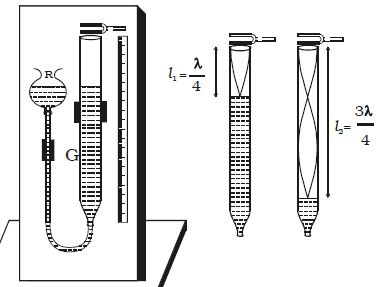Physics

# Explain Resonance Air Column Apparatus

The resonance air column apparatus consists of a glass tube G about one metre in length (Figure) whose lower end is connected to a reservoir R by a rubber tube. The glass tube is mounted on a vertical stand with a scale attached to it. The glass tube is partly filled with water. The level of water in the tube can be adjusted by raising or lowering the reservoir.A vibrating tuning fork of frequency n is held near the open end of the tube. The length of the air column is adjusted by changing the water level. The air column of the tube acts like a closed organ pipe. When this air column resonates with the frequency of the fork the intensity of sound is maximum.

Here longitudinal stationary wave is formed with node at the water surface and an antinode near the open end. If L1 is the length of the resonating air column

λ/4 = L1 + e … ….(1)

where e is the end correction.

The length of air column is increased until it resonates again with the tuning fork. If L2 is the length of the air column.

3λ/4 = L2 + e … … (2)

From equations (1) and (2)

λ/2 = (L2 – L1)

The velocity of sound in air at room temperature, v = nλ = 2n (L2 – L1)